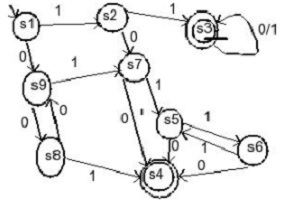# Design DFA for language over {0,1} accepting strings with odd number of 1’s and even number of 0’s

A Deterministic Finite automaton (DFA) is a 5-tuples

M=(Q, Σ, δ,q0,F)

Where,

• Q : Finite set called states.
• Σ : Finite set called alphabets.
• δ : Q × Σ → Q is the transition function.
• q0 ϵ Q is the start or initial state.
• F : Final or accept state.

## Problem

Construct a DFA machine accepting odd numbers of 1’s and even number of 0’s.

## Solution

Design two separate machines for the two conditions over an alphabet Σ={0,1}:

• DFA accepts only an odd number of 1’s.

• DFA accepts only even number of 0’s.Here,

s1 = start

s2=odd 1 or start 11

s3= starts 11 accepted and stay there

s4 = accept even 0, odd 1 and 0 1 0

s5=even 1, odd 0 and 0 1

s6=odd 1 odd 0

s7= odd 1 odd 0 start 010

s8=even 0 no 1

s0= odd 0 no 1